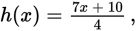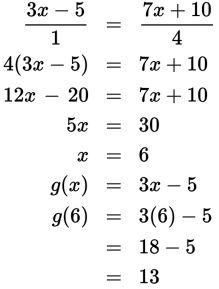# SAT Math Multiple Choice Question 248: Answer and Explanation

### Test Information

Question: 248

8. If g(x) = 3x - 5 andat what point does the graph of g(x) intersect the graph of h(x)?

• A. (-2, -11)
• B. (2, 1)
• C. (3, 4)
• D. (6, 13)

Explanation:

D

Difficulty: Medium

Category: Heart of Algebra / Systems of Linear Equations

Strategic Advice: Although this question asks where the graphs of the functions intersect, it is not necessary to actually graph them. Understanding the connection between graphing the equations and the algebra behind the graphs will save valuable time on Test Day.

Getting to the Answer: Two graphs intersect at the point where they have the same x-value and the same y-value. The notations g(x) and h(x) can both be interpreted as "the y-value at a given value of x," so set g(x) equal to h(x) and solve for x. Then plug this value into either function to find the corresponding y-value. Don't let the fraction intimidate you-you can write g(x) as a fraction over 1 and use cross-multiplication.The graphs of the functions will intersect at (6, 13).